## 2.1

• 保真度（Fidelity）：信号处理前后的相似度。
• 质量（Quality）：对图片或音频直观上的绝对评价。（看起来好或坏）

## 2.2

1. 准备

在正式测试之前，评价员将被告知测试的目的和方法，并被要求依照图像检索的场景来熟悉和理解规定的评分等级。

2. 训练

• 示例程序被用来让评价员熟悉测试程序的流程
• 在示例程序中，5个不同的参考图和5个不同强度攻击的目标图被按照正式测试的逻辑呈现，并要求评价员按照正式测试来给出评分
• 评价员在示例程序中的评分不计入最终的测试结果，但可以用来确认他对试验的目的和方法有了正确的认识
• 在示例程序中出现的参考图不会在正式测试中出现。
3. 哑元测试

• 在训练结束之后，评价员被告知进入正式测试
• 但事实上，最初几个测试结果作为哑元测试，其结果并不被程序记录，只是为了让评价员适应测试的环境。
4. 正式测试

5. 评分

1. 空间频率
2. 谱频率
3. 时间频率

# 第三章 - 数字隐写技术

## 3.1

• 信息加密：保护内容，不保护行为，现代密码学可以保证。
• 信息隐藏：信息隐蔽存在，行为也需要隐蔽，隐藏的信息容量有限。信息隐藏包括信息隐写和数字水印。

## 3.2

BMP图像文件的基本结构分为哪几个部分？各部分功能是什么？

• 位图文件头：文件类型、文件大小、偏移字节
• 位图信息头：长、宽、高，以及颜色位数值等信息
• 彩色表：可选，真彩色图（24位BMP）不需要调色板。
• 位图数据：24位图直接用RGB，其他用调色板索引。

## 3.4

LSB隐写方法的规则是什么？

1. 如果秘密信息与最低比特位相同，则不改动
2. 如果秘密信息与最低比特位不同，则使用秘密信息值代替最低比特位

LSB隐写方法的优缺点是什么？

1. 简单而又有效
2. 容量大
3. 对载体图像质量影响小
4. 嵌入速度快

## 3.6

LSB隐写方法的评价指标是什么？

• PSNR（峰值信噪比）
• 嵌入容量（$M \times N$
• 嵌入率（$\frac{1}{8}$
• 安全性

# 第四章 - 面向 JPEG 压缩的隐写方法

## 4.1

JPEG图像与BMP图像相比较的优缺点是什么？

JPEG 存储空间小、需要处理，BMP 质量好。

## 4.2

DCT变换前图像进行哪些预处理，目的是什么？

1. 分块：只有比较小的邻域内的像素有比较高的相关性，相距远的话，其相关性就大大下降。
2. 将 RGB 转换为 YCbCr：把重要的信息和不重要的信息分开。对于人眼来说，图像中明暗的变化更容易被感知到，这是由于人眼的构造引起的。

## 4.3

JPEG压缩分为哪几个步骤？哪些位置适合隐写，为什么？

1. 分块
2. 转换为 YCbCr
3. 正向 DCT 变换
4. 量化
5. 熵编码

## 4.4

JPEG压缩过程中使用DCT变换的目的是什么？

## 4.5

Jsteg隐写是将秘密信息嵌入在量化后的DCT系数的LSB上，但原始值为（-1,0，+1）的DCT系数除外。

F3隐写对于非0的DCT数据用于隐藏1比特秘密信息，为0的DCT系数不负载秘密信息。（隐写数量增加）

## 4.6

$\begin{pmatrix} 97 & 0 & -1 & -1 & 0 & 0 & 0 & 0 \\ -3 & -2 & 4 & 0 & 0 & 0 & 0 & 0 \\ -2 & -1 & 0 & 0 & 0 & 0 & 0 & 0 \\ \cdots \end{pmatrix}$

Jsteg：

1. 0: 97 -> 96
2. 0: -3 -> -2
3. 1: -2 -> -3
4. 1: 4 -> 5
5. 0: -2 -> -2

$\begin{pmatrix} 96 & 0 & -1 & -1 & 0 & 0 & 0 & 0 \\ -2 & -3 & 5 & 0 & 0 & 0 & 0 & 0 \\ -2 & -1 & 0 & 0 & 0 & 0 & 0 & 0 \\ \cdots \end{pmatrix}$

F3:

1. 0: 97 -> 96
2. 0: -1 -> 0, -1 -> 0, -3 -> -2
3. 1: -2 -> -3
4. 1: 4 -> 5
5. 0: -2 -> -2

$\begin{pmatrix} 96 & 0 & 0 & 0 & 0 & 0 & 0 & 0 \\ -2 & -3 & 5 & 0 & 0 & 0 & 0 & 0 \\ -2 & -1 & 0 & 0 & 0 & 0 & 0 & 0 \\ \cdots \end{pmatrix}$

# 第六章 - 数字图像隐写技术

## 6.2

$C_{max}$ = 7（每行7对） * 8（共8行） * 2（行、列均考虑） = 112

## 6.3

$a<0.5$，如果原来的复杂度小于 $aC_{max}$，经过共轭处理之后复杂度变为 $(1-a)C_{max}$，满足大于 $aC_{max}$ 的条件，适合进行隐写。

## 6.4

1. 分块（$8\times 8$
2. 计算每个小块的复杂度（$C$
3. 将复杂度大于 $aC_{max}, a < 0.5$ 的平面块用于负载秘密信息
4. 将秘密信息构成同样大小的块，如果秘密信息的复杂度小于 $aC_{max}$，则做共轭处理之后再替换
5. 记录下经过共轭处理的小块信息（位置、位平面）

## 6.5

1. 记录小块中像素灰度差值

$d = p_{i+1}-p_i=113-105=8$

2. 将亮度范围分成 $K$ 个区域，每个区域的宽度都是 2 的整数幂。

$[0,7],[8,15],[16,31],[32,63],[64,127],[128,255]$

3. 记每个区域的下界、上界和宽度为 $l_k, u_k, w_k$

4. 如果 $|d|$ 落在区域 $R_k$ 中，则这个小块中嵌入 $\log_2 w_k$ 个秘密信息比特。

$d=8 \in [8, 15]$，在这个小块中嵌入 $\log_2 8 = 3$，嵌如 1, 1, 1。

5. $\log_2 w_k$ 个秘密信息比特转化为十进制 $b$，并计算。

$b = 111_2 = 7$

因为 $d > 0$，所有 $d' = l_k+b=8+7=15$

6. 嵌入计算

$d \% 2 = 0$

$r_c = \lceil \frac{15-8}{2} \rceil = 4, r_f = \lfloor \frac{15-8}{2}=3 \rfloor$

$(p'_i, p'_{i+1}) = (p_i - r_f, p_{i+1}+r_c) = (105-3, 113+4) = (102, 117)$

$d' = 117-102=15$

$d' \in [8, 15]$

$b = |d'| - l_k = 15 - 8 = 7$

$\log_2 8 = 3$ 位秘密信息，为 $111_2$

$d = 101 - 118 = -17 \\ [16, 31] \\ \log_2 16 = 4 位秘密信息 \\ 0101_4 = 5 \\ d' = -(l_k + b) = -(16 + 5) = -21 \\ d\%2 = 1 \\ r_c = \lceil \frac{-21-(-17)}{2} \rceil = -2, r_f = \lfloor \frac{-21-(-17)}{2} \rfloor = -2 \\ (p'_i, p'_{i+1}) = (p_i - r_c, p_{i+1}+r_f) = (118-(-2), 101+(-2)) = (120, 99) \\$

$d' = 99 - 120 = -21 \in [16, 31] \\ b = |d'| - l_k = 21 - 16 = 5 \\ \log_2 16 = 4 位秘密信息，为 0101_2 \\$

# 第七章 - 隐写分析技术

## 7.3

2i <-> 2i+1

2i-1 <-> 2i

0 翻转 $F_0$

## 7.4

1. 分块

2. 对每块进行非负、非正翻转

3. 计算每块图像的像素相关性是否增加

$f = \sum_{x 按照 Z 排序 } |x_i - x_{i-1}|$

4. 将非负翻转后像素相关性增加的图像块的比例记为 $R_m$，相关性减小的图像的比例记为 $S_m$

5. 将非正翻转后像素相关性增加的图像块的比例记为 $R_{-m}$，相关性减小的图像的比例记为 $S_{-m}$

$R_m \approx R_{-m} \\ S_m \approx S_{-m}$

$R_m > S_m \\ R_{-m} > S_{-m}$

## 7.5

1. 未隐写时，DCT 系数服从 Laplace 分布
2. 隐写之后，DCT 系数不再服从 Laplace 分布，利用分布拟合，给定置信区间，判断是否符合 Laplace 分布。

# 第八章 - 抗隐写分析的 LSB 和 JPEG 隐写

## 8.1

$f_{2i+1}$ 中未嵌入的像素来补偿 $f_{2i}$ 中所有嵌入成 $f_{2i+1}$ 的像素：

$(1-\alpha)f_{2i+1} \ge \frac{\alpha}{2}(f_{2i} - f_{2i+1})$

1. 统计原始图像的灰度直方图，确定 $f_{2i}, f_{2i+1}$
2. 计算秘密信息隐藏率 $\alpha$
3. 将所有的像素值排成一个向量，长度为 $l$
4. 秘密信息嵌入到 $\lfloor \alpha \times l \rfloor$ 为止
5. 如果 $f_{2i}$ 变大，将 $\lfloor \alpha \times l \rfloor + 1$ 之后的像素中的值为 $2i$ 的像素变为 2i+1，尽可能保持直方图不发生变化；反之类似。

## 8.2

$\alpha \le \frac{2f_{2i+1}}{f_{2i}+f_{2i+1}}$

## 8.3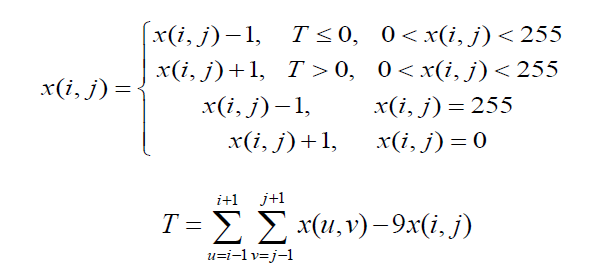$T$$x(i, j)$ 处于边界时无法计算，这是可以采用其他方法给边界插值，特殊情况，对总体的影响不大。

## 8.4

• 255 翻转只能变成 254
• 0 翻转只能变成 1

## 8.5

F5 隐写算法使用了哪两种重要方法，其目的是什么？

• 混洗技术：使秘密信息分布在整幅图像中
• 矩阵编码：是为了提高嵌入效率。

## 8.6

F5 隐写后图像的哪种统计特性发生了变化？

• 块边界的不连续性增加，$k$ 越小，现象越明显。

## 8.7

$x_1 = a_1 \oplus a_4 \oplus a_5 \oplus a_7 \\ x_2 = a_2 \oplus a_4 \oplus a_6 \oplus a_7 \\ x_3 = a_3 \oplus a_5 \oplus a_6 \oplus a_7 \\$

\begin{aligned} x_1, x_2, x_3 &= 1, 0, 1 \\ a_1, \cdots, a_7 &= 13, 17, 16, 16, 19, 21, 20 = 1, 1, 0, 0, 1, 1, 0 \end{aligned}

$1 \neq 1 \oplus 0 \oplus 1 \oplus 0 \\ 0 = 1 \oplus 0 \oplus 1 \oplus 0 \\ 1 \neq 0 \oplus 1 \oplus 1 \oplus 0 \\$

# 第九章 - 调色板图像隐写

## 9.1

1. 不超过 256 种颜色的调色板，定义每种颜色的 RGB 各颜色分量值。
2. 图像内容，其中每个像素是一个不超过 $8$ 位的索引值，指向调色板中的对应颜色。

## 9.2

$\lfloor \log_2(M!) \rfloor$ 位秘密信息。

## 9.3

1. 将真实颜色进行排序
2. 秘密信息变为十进制：$1010 \to 10$
3. 查询密码本对应的序列：$10$ 对应的序列为 $1, 3, 2, 4$
4. 改变图像内容中索引顺序为 $1, 3, 2, 4$

## 9.5

1. 将调色板的颜色亮度 （Y）依次排序
2. 为每个颜色分配一个亮度序号，然后用奇数序号表示嵌入秘密比特$1$，用偶数序号表示嵌入秘密比特 $0$
3. 将调色板图像像素内容（索引值）使用 LSB 隐写代替，改为新的亮度序号所对应的的索引值。

## 9.6

（对于EZStego算法）

• $[0, 20) \to 0$
• $[20, 40) \to 20$
• $[40, 60) \to 40$
• $\cdots$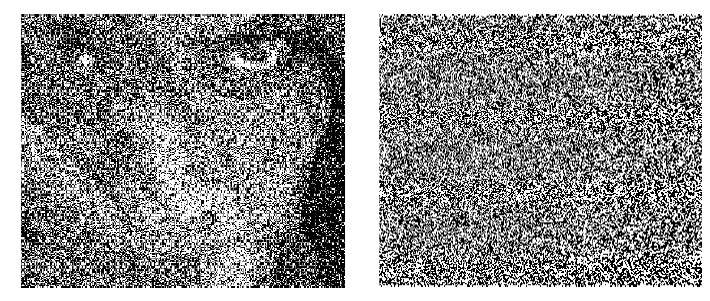## 9.7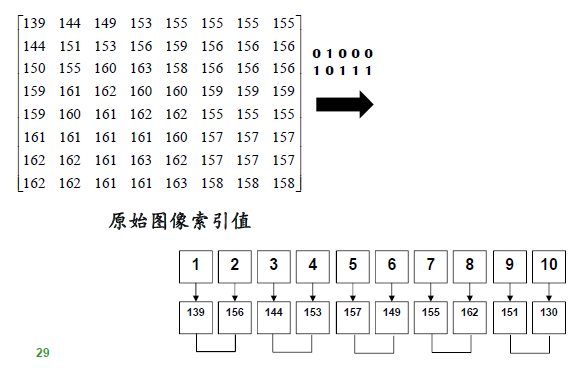# 第十章 - 数字水印技术

• 所有者鉴别
• 所有权验证
• 操作跟踪
• 拷贝控制

• 完整性认证
• 篡改监测

## 10.3

$X' = X + (\alpha b - \lambda s')\omega$

• $X'$: 含水印图像
• $X$: 原始图像
• $\alpha$: 嵌入强度
• $b$: 水印信息
• $\lambda$: 常数，值为 $1$
• $s'$: 原始图像与扩频序列的相关性
• $\omega$: 扩频序列

$s' = \frac{1}{N}\sum_{i=1}^N X_i \omega_i$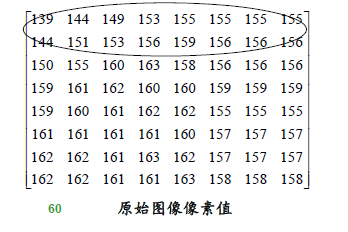• 水印信息：$-1, 1, -1, 1$
• 扩频序列：$1 \to 1, -1, -1, 1; -1 \to -1, 1, 1, -1$
• 扩频水印：（自己扩一下）

\begin{aligned} s' &= \frac{1}{4}(-1\times 139 +1\times 144 + 1 \times 149 + (-1) \times 153) \\ &= \frac{1}{4} \end{aligned}

\begin{aligned} X' &= X + (\alpha b - \lambda s')\omega \\ &= (139, 144, 149, 153) + (-1, 1, 1, -1) \times (4 \times (-1) - 1 \times \frac{1}{4}) \\ &= (139, 144, 149, 153) + (4.25, -4.25, -4.25, 4.25) \\ &= (143.25, 139.75, 144.75, 157.25) \\ &= (143, 140, 145, 157) \end{aligned}

## 10.4

$cor(X', \omega) = \alpha b$

\begin{aligned} \alpha b &= cor(X', \omega) \\ &= \frac{1}{4} (143, 140, 145, 157) \cdot (-1, 1, 1, -1) \\ &= \frac{1}{4} \times (-15) \\ &= -4 \end{aligned}

# 第十一章 - 空域数字图像鲁棒水印

## 11.3

• $I_{low}$：宿主图像的低频部分
• $H$：不同灰度级下的像素个数

## 11.4

1. 高斯滤波
2. 抽取直方图，如上一题所述。
3. 像素分组，将 $L_G$ 个相邻灰度级下的像素分到同一个分组中，这样我们就会得到 $M_G = \lfloor M/L_G \rfloor$ 个像素分组。第 $i$ 个分组中有 $h_G(i)$ 个像素。每组分为$B_1$$B_2$两部分。
4. 水印嵌入，修改 Bin1，Bin2 中的像素，将 Bin1 中的像素落入 Bin2 中的灰度值，或者 Bin2 中的像素落入到 Bin1 中的灰度值，从而使得 $\frac{h'_{B1}(i)}{h'_{B2}(i)} = \lambda$（嵌入 $1$ 的话是 $\lambda$$0$$\frac{1}{\lambda}$

## 11.6

1. 判断像素值的峰值点（61）和零点（64，必须有零点，不然无法弄）
2. 将峰值点与零点的像素平移成相邻位置（$63 \to 64, 62 \to 63$
3. 向峰值像素值中嵌入水印信息，$1$ 不变，$0$ 变为零点值（$61 \to 62$）。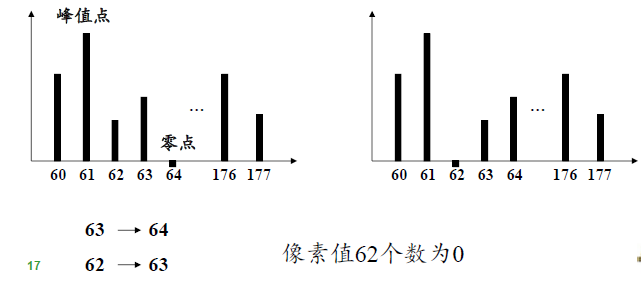1. 从像素值为 $61$$62$ 的像素值之中提取水印，看到 $61$ 提取水印 $1$，看到 $62$ 提取水印 $0$，同时将 $62$ 变为 $61$
2. 平移修改的像素 $63 \to 62, 64 \to 63$

1. 最近邻插值法
2. 双线性插值法
3. 双三插值法

## 11.10

1. 图像分块

2. 对每个分块进行 DFT 变换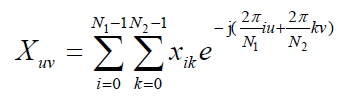3. 可以通过适当修改 $DFT$ 参数的幅度 $|X_{uv}|$ 进行水印嵌入。

修改幅度满足对称条件：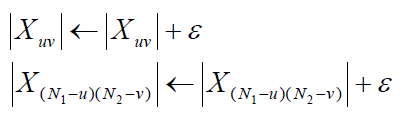# 第十二章 - DWT(小波) 变换域数字水印嵌入技术

## 12.2

1. 将灰度值分为两个区间：比如区间 1 为 $[0, 128]$，区间 2 为 $[129, 255]$，分别对应水印 $0$ 和 水印 $1$

2. 根据水印和像素值，根据水印信息变为对应区间中的一个随机值，计算含水印像素值

• 水印：$[0, 1, 0, 1]$
• 像素值：$[190, 12, 66, 200]$
• 含水印像素值：$[64, 192, 63, 190]$